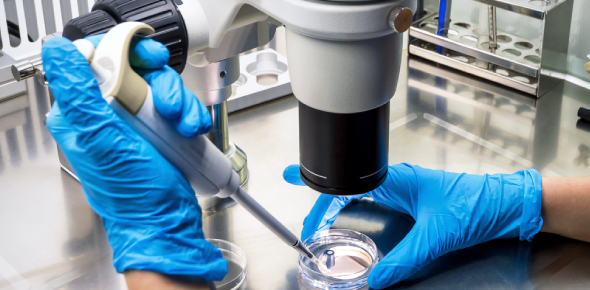# Microbiology Chapter 2

19 Questions | Total Attempts: 1346SettingsQuiz on Chapter 2 Microbio

Related Topics
• 1.
Which is the smallest chemical unit of matter?
• A.

An element.

• B.

A molecule.

• C.

An atom.

• D.

Carbon (C)

• E.

None of the above

• 2.
CO2 is an example of a
• A.

Atom.

• B.

Compound.

• C.

electron.

• D.

Element.

• E.

None of the above.

• 3.
How many electrons can fit on the 2nd shell of an element?
• A.

2

• B.

4

• C.

6

• D.

8

• E.

18

• 4.
If an atom looses an electron it has an overall __________ charge.
• A.

Negative

• B.

Neutral

• C.

Positive

• D.

None of the above.

• 5.
Which ring of electrons would have the highest energy level?
• A.

The first ring.

• B.

The last ring.

• C.

The second ring.

• D.

They are all the same.

• 6.
The nucleus of every atom bears a net _______ charge.
• A.

Positive

• B.

Negative

• C.

Neutral

• D.

None of the above.

• 7.
An atom that looses an electron is called a ________, while an atom that gains an electron is called a _______.
• A.

Nucleus, electron

• B.

Anion, Cation

• C.

Element, molecule

• D.

Cation, Anion

• 8.
A bond where pairs of electrons are shared in order to become stable is called
• A.

A hydrogen bond

• B.

A covalent bond

• C.

An ionic bond.

• D.

James bond.

• E.

Polar bond.

• 9.
A hydrogen bond is the strongest of all bonds.
• A.

True

• B.

False

• 10.
A bond between a cation and an anion is called a
• A.

Ionic bond

• B.

Covalent bond.

• C.

Hydrogen bond

• D.

Polar bond

• 11.
Hydrogen bonds bind atoms into molecules.
• A.

True

• B.

False

• 12.
The attraction between oppositely charged regions of different molecules is called a ________ bond.
• A.

Hydrogen

• B.

Ionic

• C.

Polar

• D.

Covalent

• 13.
There are 4 main reasons that water is highly useful for living things, select all correct answers.
• A.

Every molecule can form 4 hydrogen bonds with other water molecules.

• B.

Water changes temperature very easily.

• C.

The polarity of water makes it an excellent solvent

• D.

Since water is extremely combustible it has many uses for living things.

• E.

None of the above are correct.

• 14.
In order for a compound to be considered organic it must contain these two elements.
• A.

Sulfur and nitrogen

• B.

Hydrogen and water

• C.

Carbon and oxygen

• D.

Hydrogen and carbon

• E.

Proteins and carbohydrates.

• 15.
Monosaccharides, Disaccharides and Polysaccharides are the major groups of _________.
• A.

Nucleic acids

• B.

Proteins

• C.

Lipids

• D.

Carbohydrates

• 16.
Starch is an example of a
• A.

Polysaccharide

• B.

Monosaccharide

• C.

Protein

• D.

Nucleic acid

• 17.
Hundreds of monosaccharides joined together is called a __________.
• A.

Microorganism

• B.

Polysaccharide

• C.

Bacteria

• D.

Protein

• E.

Two of the above

• 18.
In order to be called a carbohydrate, the compound must include these three elements.
• A.

C, H, and O

• B.

N, H, and O

• C.

S, N and Na

• D.

None of the above are correct.

• 19.
______ are essential to the structure and function of membranes.
• A.

Proteins

• B.

Monosaccharides

• C.

Lipids

• D.

Nucleic Acids

• E.

Chuck Norris